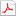# ASSVD: Adaptive Sparse Singular Value Decomposition for High Dimensional Matrices

최초 등록일
2020.07.28
최종 저작일
2020.07
15페이지/어도비 PDF
가격
판매자한국학술정보(주)
다운로드
장바구니

* 본 문서는 배포용으로 복사 및 편집이 불가합니다.

## 서지정보

발행기관 : 한국인터넷정보학회 수록지정보 : KSII Transactions on Internet and Information Systems (TIIS) / 14권 / 6호
저자명 : ( Xiucai Ding ) , ( Xianyi Chen ) , ( Mengling Zou ) , ( Guangxing Zhang )

## 영어 초록

In this paper, an adaptive sparse singular value decomposition (ASSVD) algorithm is proposed to estimate the signal matrix when only one data matrix is observed and there is high dimensional white noise, in which we assume that the signal matrix is low-rank and has sparse singular vectors, i.e. it is a simultaneously low-rank and sparse matrix. It is a structured matrix since the non-zero entries are confined on some small blocks. The proposed algorithm estimates the singular values and vectors separable by exploring the structure of singular vectors, in which the recent developments in Random Matrix Theory known as anisotropic Marchenko-Pastur law are used. And then we prove that when the signal is strong in the sense that the signal to noise ratio is above some threshold, our estimator is consistent and outperforms over many state-of-the-art algorithms. Moreover, our estimator is adaptive to the data set and does not require the variance of the noise to be known or estimated. Numerical simulations indicate that ASSVD still works well when the signal matrix is not very sparse.

없음

더보기 (5/10)

## 자료문의

제휴사는 별도로 자료문의를 받지 않고 있습니다.

## 판매자 정보한국학술정보(주)는 콘텐츠 제작에 도움이 되는 솔루션을 기반으로 풍부한 문화 콘텐츠를 생성하여 새로운 삶의 가치를 창조합니다.

본 학술논문은 한국학술정보(주)와 각 학회간에 저작권계약이 체결된 것으로 AgentSoft가 제공 하고 있습니다.
본 저작물을 불법적으로 이용시는 법적인 제재가 가해질 수 있습니다.최근 본 자료더보기